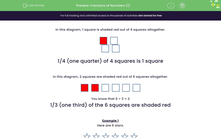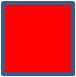# Find Simple Fractions of Numbers Using Pictures

In this worksheet, students will find simple fractions of numbers using pictures.Key stage:  KS 1

Curriculum topic:   Number: Fractions

Curriculum subtopic:   Write Fractions

Popular topics:   Fractions worksheets

Difficulty level:#### Worksheet Overview

In this activity, we are going to be finding simple fractions.

In this diagram, 1 square is shaded red out of 4 squares altogether. So 1 out of 4 squares is red. We write this as 1/4 or one quarter of the squares are red.1/4 (one quarter) of 4 squares is 1 square

In this diagram, 2 squares are shaded red out of 6 squares altogether. So, 2 out of 6 are shaded red. We write this as 2/6.We know that 2/6 of the squares are red, but we can simplify this fraction to make an equivalent one.

We can divide both the top number (2) and the bottom number (6) by the number 2 to make an equivalent fraction:

2 ÷ 2 = 1
6 ÷ 2 = 3

So 2/6 is the same as 1/3

1/3 (one third) of the 6 squares are shaded red

Example 1

Here are 6 stars.Anna colours ½ of them red.

How many does she colour red?

There are 6 stars in total

6 ÷ 2 = 3

She colours 3 of them red.½ (one half) of the stars are shaded red.

½ of 6 is 3

Example 2

Here are 6 triangles.Anna colours 1/3 of them red.

How many does she colour red?

There are 6 triangles

To find one third of them, we need to divide by 3:

6 ÷ 3 = 2

She colours 2 of them red.1/3 (one third) of the triangles are shaded red.

1/3 of 6 is 2

Now it's your turn to try some questions.

### What is EdPlace?

We're your National Curriculum aligned online education content provider helping each child succeed in English, maths and science from year 1 to GCSE. With an EdPlace account you’ll be able to track and measure progress, helping each child achieve their best. We build confidence and attainment by personalising each child’s learning at a level that suits them.

Get started